# Texas Go Math Grade 6 Lesson 16.2 Answer Key Area of Triangles

Refer to our Texas Go Math Grade 6 Answer Key Pdf to score good marks in the exams. Test yourself by practicing the problems from Texas Go Math Grade 6 Lesson 16.2 Answer Key Area of Triangles.

## Texas Go Math Grade 6 Lesson 16.2 Answer Key Area of Triangles

Explore Activity 1

Area of a Right Triangle
A. Draw a large rectangle on grid paper.
What is the formula for the area of a rectangle? A = ____________B. Draw one diagonal of your rectangle.
The diagonal divides the rectangle into ____________.
Each one represents ____________ of the rectangle.
Use this information and the formula for area of a rectangle to write a formula for the area of a right triangle. A = ____________

Reflect

Question 1.
Communicate Mathematical Ideas In the formula for the area of a right triangle, what do b and h represent?
b is the base of the right triangle and h is the height of the right triangle.

Explore Activity 2

Area of a Triangle

A. Draw a large triangle on grid paper. Do not draw a right triangle.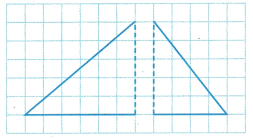B. Cut out your triangle. Then trace around it to make a copy of your triangle. Cut out the copy.
C. Cut one of your triangles into two pieces by cutting through one angle directly across to the opposite side. Now you have three triangles — one large triangle and two smaller triangles.
When added together, the areas of the two smaller triangles equal the __________ of the large triangle.
D. Arrange the three triangles into a rectangle.What fraction of the rectangle does the large triangle represent? __________
The area of the rectangle is A = bh. What is the area of the large triangle? A = __________
How does this formula compare to the formula for the area of a right triangle that you found in Explore Activity 1 ? __________

Reflect

Question 2.
Communicate Mathematical Ideas What type of angle is formed by the base and height of a triangle?
The height of a triangle is always the perpendicular distance between the base and its top edge, so it can be said that the angle between the base and height of a triangle is always 90°.

Find the area of the triangle.

Question 3.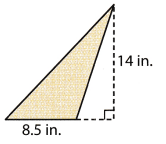A = _____________
Find the area of the triangle.
b = 8.5 inches h = 14 inches
A = $$\frac{1}{2}$$ bh
= $$\frac{1}{2}$$ (8.5 inches)(14 inches) Substitute
= 59.5 square inches Multiply
= 59.5 in2

Question 4.
Amy needs to order a shade for a triangular-shaped window that has a base of 6 feet and a height of 4 feet. What is the area of the shade?
Data:
b = 6
h = 4
Write equation of area of a triangle:
Area = $$\frac{1}{2}$$ × b × h
Substitute values:
Area = $$\frac{1}{2}$$ × 6 × 4
Evaluate:
Area = 12
Area of the shade is 12 square feet.

Question 1.
Show how you can use a copy of the triangle to form a rectangle. Find the area of the triangle and the area of the rectangle. What is the relationship between the areas? (Explore Activities 1 and 2, Example 1)Transforming the triangle to rectangle.Determine the area of the triangle.
A = $$\frac{1}{2}$$ (14) (8) substitute for the area of the triangle
A = $$\frac{1}{2}$$ (112) simplify
A = 56 sq. in. area of the triangle
A = 14 × 8 substitute for the area of the rectangle
A = 112 sq. in. area of the rectangle
The area of the triangle is 56 sq. in. while the area of the rectangle is 112 sq. in. which shows that the area of the triangle is half of the area of the rectangle.

Question 2.
A pennant in the shape of a triangle has a base of 12 inches and a height of 30 inches. What is the area of the pennant? (Example 2)A = $$\frac{1}{2}$$bh
= $$\frac{1}{2}$$ (________) (_______)
= ________ in2
Data:
b = 12
h = 30
Write equation of area of a triangle:
Area = $$\frac{1}{2}$$ × b × h
Substitute values:
Area = $$\frac{1}{2}$$ × 12 × 30
Evaluate:
Area = 180
Area of the pennant is 180 square inches.

Essential Question Check-In

Question 3.
How do you find the area of a triangle?
Area of a triangle is the product of its height and base divided by 2. Mathematically the formula for area of a triangle is:
Area = $$\frac{1}{2}$$ × b × h

Find the area of each triangle.

Question 4.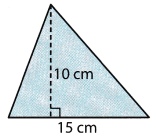Find the area of the triangle.
b = 15 cm h = 10 cm
A = $$\frac{1}{2}$$bh
= $$\frac{1}{2}$$ (15 cm) (10 cm) Substitute
= 75 square centimeters Multiply
= 75 cm2

Question 5.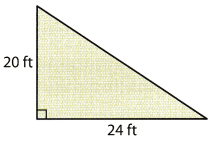Find the area of the triangle.
b = 24 feet; h = 20 feet
A = $$\frac{1}{2}$$bh
= $$\frac{1}{2}$$ (24 feet) (20 feet) Substitute
= 240 square feet Multiply
= 240 ft2

Question 6.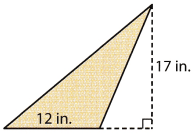Find the area of the triangle.
b = 12 inches; h = 17 inches
A = $$\frac{1}{2}$$bh
= $$\frac{1}{2}$$ (12 inches) (17 inches) Substitute
= 102 square inches Multiply
= 102 in2

Question 7.Find the area of the triangle.
b = 18 feet; h = 32 feet
A = $$\frac{1}{2}$$bh
= $$\frac{1}{2}$$ (18 feet) (32 feet) Substitute
= 288 square feet Multiply
= 288 ft2

Question 8.
What is the area of a triangle that has a base of 15$$\frac{1}{4}$$ in. and a height of 18 in.?
Solution to this example is given below
$$15 \frac{1}{4}=\frac{15 \times 4+1}{4}=\frac{61}{4}$$ = 15.25 Convert to decimal number
Find the area of the triangle.
b = 15.25 inches; h = 18 inches
A = $$\frac{1}{2}$$bh
= $$\frac{1}{2}$$ (15.25 inches) (18 inches) Substitute
= 137.25 square inches Multiply
= 137.25 in2

Question 9.
A right triangle has legs that are 11 in. and 13 in. long. What is the area of the triangle?
Find the area of the triangle
b = 11 inches; h = 13 inches
A = $$\frac{1}{2}$$bh
= $$\frac{1}{2}$$ (11 inches) (13 inches) Substitute
= 71.5 square inches Multiply
= 71.5 in2

Question 10.
A triangular plot of land has the dimensions shown in the diagram. What is the area of the land?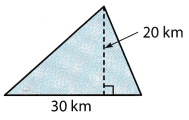Find the area of the triangle.
b = 30km; h = 20 km
A = $$\frac{1}{2}$$bh
= $$\frac{1}{2}$$ (30 km) (20 km) Substitute
= 300 square kilometer Multiply
= 300 km2

Question 11.
The front part of a tent has the dimensions shown in the diagram. What is the area of this part of the tent?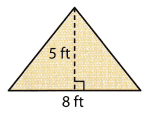Data:
b = 8
h = 5
Write equation of area of a triangle:
Area = $$\frac{1}{2}$$ × b × h
Substitute values:
Area = $$\frac{1}{2}$$ × 8 × 5
Evaluate:
Area = 20
Area of the front of the tent is 20 square feet.

Question 12.
Multistep The sixth-grade art students are making a mosaic using tiles in the shape of right triangles. Each tile has leg measures of 3 centimeters and 5 centimeters. If there are 200 tiles in the mosaic, what is the area of the mosaic?
Data:
b = 3
h = 5
Write equation of area of a triangle:
Area = $$\frac{1}{2}$$ × b × h
Substitute values:
Area = $$\frac{1}{2}$$ × 3 × 5
Evaluate:
Area = 7.5
The area of 1 tile is 7.5 square centimeters so the total area of 200 tiles is 7.5 × 200 = 1500 square centimeters.
Total area of 200 tiles is 1500 square centimeters.

Question 13.
Critique Reasoning Monica has a triangular piece of fabric. The height of the triangle is 15 inches and the triangle’s base is 6 inches. Monica says that the area of the fabric is 90 in2. What error did Monica make? Explain your answer.
Find the area of the triangle
b = 6 inches h = 15 inches
A = $$\frac{1}{2}$$bh
= $$\frac{1}{2}$$ (6 inches) (15 inches) Substitute
= 45 square inches Multiply
Area of the piece of cloth is 45 square inches.
= 45 in2

Question 14.
Show how you can use the given triangle and its two smaller right triangles to form a rectangle. What is the relationship between the area of the original triangle and the area of the rectangle?Transform the triangle together with its two smaller triangles to form a rectangle.Determine the area of the triangle.
A = $$\frac{1}{2}$$ (18) (12) substitute for the area of the triangle
A = $$\frac{1}{2}$$ (216) simplify
A = 108 sq. in. area of the triangle
A = 18 × 12 substitute for the area of the rectangle
A = 216 sq. in. area of the rectangle
The area of the triangle is 108 sq. in. while the area of the rectangle is 216 sq. in. which shows that the area of the rectangle is twice the area of the triangle.

H.O.T. Focus on Higher Order Thinking

Question 15.
Communicate Mathematical Ideas Explain how the areas of a triangle and a parallelogram with the same base and height are related.
The area of a parallelogram with a base b and height h is always twice that of a triangle with the same dimensions. This is because this parallelogram when cut along its diagonal, gives 2 triangles with the same dimensions but half the area as that of the parallelogram.

Question 16.
Analyze Relationships A rectangle and a triangle have the same area. If their bases are the same lengths, how do their heights compare? Justify your answer.
The area of a rectangle with a length b and height h is always twice that of a triangle with the same dimensions. If their areas and their bases are same, then this implies that the height of the rectangle wilt be half of that of the triangle.

Question 17.
What If? A right triangle has an area of 18 square inches.
a. If the triangle is an isosceles triangle, what are the lengths of the legs of the triangle?
Data:
Area = 18
l = b = x
Write equation of area of a triangle:
Area = $$\frac{1}{2}$$ × b × h
Substitute values:
18 = $$\frac{1}{2}$$ × x × x
Solve for x:
x2 = 18 × 2
Apply square root on both sides of the equation:
Evaluate:
x = 6
The base and height of the isosceles triangle is equal to 6 inches.

b. If the triangle is not an isosceles triangle, what are all the possible lengths of the legs, if the lengths are whole numbers?